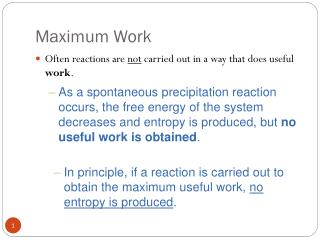DownloadDownload PresentationMaximum Work

# Maximum Work

Download Presentation## Maximum Work

- - - - - - - - - - - - - - - - - - - - - - - - - - - E N D - - - - - - - - - - - - - - - - - - - - - - - - - - -
##### Presentation Transcript

1. Maximum Work • Often reactions are not carried out in a way that does useful work. • As a spontaneous precipitation reaction occurs, the free energy of the system decreases and entropy is produced, but no useful work is obtained. • In principle, if a reaction is carried out to obtain the maximum useful work, no entropy is produced.

2. Maximum Work • Often reactions are not carried out in a way that does useful work. • It can be shown that the maximum useful work,wmax , for a spontaneous reaction isDG. • The term free energy comes from this result.

3. Free Energy Change During Reaction • As a system approaches equilibrium, the instantaneous change in free energy approaches zero. • Figure 19.9 illustrates the change in free energy during a spontaneous reaction. • As the reaction proceeds, the free energy eventually reaches its minimum value. • At that point,DG= 0, and the net reaction stops; it comes to equilibrium.

4. Relating DGo to the Equilibrium Constant The free energy change when reactants are in non-standard states (other than 1 atm pressure or 1 M) is related to the standard free energy change, DGo, by the following equation. • Here Q is the thermodynamic form of the reaction quotient.

5. Relating DGo to the Equilibrium Constant • The free energy change when reactants are in non-standard states (other than 1 atm pressure or 1 M) is related to the standard free energy change, DGo, by the following equation. • DGrepresents an instantaneous change in free energy at some point in the reaction approaching equilibrium.

6. Relating DGo to the Equilibrium Constant • The free energy change when reactants are in non-standard states (other than 1 atm pressure or 1 M) is related to the standard free energy change, DGo, by the following equation. • At equilibrium,DG=0and the reaction quotient Q becomes the equilibrium constant K.

7. Relating DGo to the Equilibrium Constant • The free energy change when reactants are in non-standard states (other than 1 atm pressure or 1 M) is related to the standard free energy change, DGo, by the following equation. • At equilibrium,DG=0and the reaction quotient Q becomes the equilibrium constant K.

8. Relating DGo to the Equilibrium Constant • This result easily rearranges to give the basic equation relating the standard free-energy change to the equilibrium constant. • When K > 1 , the ln K is positive andDGois negative. • When K < 1 , the ln K is negative andDGo is positive.

9. Spontaneity and Temperature Change • All of the four possible choices of signs for DHo and DSo give different temperature behaviors for DGo.

10. You get the value ofDGToat any temperature T bysubstituting values ofDHoandDSoat 25 oC into the following equation. Calculation of DGo at Various Temperatures • In this method you assume that DHo and DSo are essentially constant with respect to temperature.

11. DHfo: -1206.9 -635.1 -393.5 kJ So: 92.9 38.2 213.7 J/K A Problem To Consider • Find the DGo for the following reaction at 25oC and 1000oC. Relate this to reaction spontaneity.